Det här är ett avsnitt i en webbkurs om databaser som finns fritt tillgänglig på adressen http://www.databasteknik.se/webbkursen/. Senaste ändring: 26 juni 2008. Av Thomas Padron-McCarthy. Copyright, alla rättigheter reserverade, osv. Skicka gärna kommentarer till webbkursen@databasteknik.se.

# Lecture Notes: Relational Algebra

Det finns inget kapitel om relationsalgebra i kursen. Jag hade först tänkt ha med ett, men relationsalgebra passar inte riktigt i en grundkurs som den här. I stället finns en kort förklaring i ordlistan, och för den som vill läsa mer finns dessutom dessa föreläsningsanteckningar på engelska.

## What? Why?

• Similar to normal algebra (as in 2+3*x-y), except we use relations as values instead of numbers, and the operations and operators are different.
• Not used as a query language in actual DBMSs. (SQL instead.)
• The inner, lower-level operations of a relational DBMS are, or are similar to, relational algebra operations. We need to know about relational algebra to understand query execution and optimization in a relational DBMS.
• Some advanced SQL queries requires explicit relational algebra operations, most commonly outer join.
• Relations are seen as sets of tuples, which means that no duplicates are allowed. SQL behaves differently in some cases. Remember the SQL keyword distinct.
• SQL is declarative, which means that you tell the DBMS what you want, but not how it is to be calculated. A C++ or Java program is procedural, which means that you have to state, step by step, exactly how the result should be calculated. Relational algebra is (more) procedural than SQL. (Actually, relational algebra is mathematical expressions.)

## Set operations

Relations in relational algebra are seen as sets of tuples, so we can use basic set operations.

## Review of concepts and operations from set theory

• set
• element
• no duplicate elements (but: multiset = bag)
• no order among the elements (but: ordered set)
• subset
• proper subset (with fewer elements)
• superset
• union
• intersection
• set difference
• cartesian product

## Projection

Example: The table E (for EMPLOYEE)

nrnamesalary
1John100
5Sarah300
7Tom100

SQLResultRelational algebra
```select salary
from E
```
salary
100
300
PROJECTsalary(E)
```select nr, salary
from E
```
nrsalary
1100
5300
7100
PROJECTnr, salary(E)

Note that there are no duplicate rows in the result.

## Selection

The same table E (for EMPLOYEE) as above.

SQLResultRelational algebra
```select *
from E
where salary < 200
```
nrnamesalary
1John100
7Tom100
SELECTsalary < 200(E)
```select *
from E
where salary < 200
and nr >= 7
```
nrnamesalary
7Tom100
SELECTsalary < 200 and nr >= 7(E)

Note that the select operation in relational algebra has nothing to do with the SQL keyword select. Selection in relational algebra returns those tuples in a relation that fulfil a condition, while the SQL keyword select means "here comes an SQL statement".

## Relational algebra expressions

SQLResultRelational algebra
```select name, salary
from E
where salary < 200
```
namesalary
John100
Tom100
PROJECTname, salary (SELECTsalary < 200(E))

or, step by step, using an intermediate result

Temp <- SELECTsalary < 200(E)
Result <- PROJECTname, salary(Temp)

## Notation

The operations have their own symbols. The symbols are hard to write in HTML that works with all browsers, so I'm writing PROJECT etc here. The real symbols:

Operation My HTML Symbol
Projection PROJECTSelection SELECTRenaming RENAME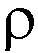Union UNIONIntersection INTERSECTION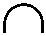Assignment <-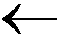Operation My HTML Symbol
Cartesian product XJoin JOINLeft outer join LEFT OUTER JOIN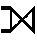Right outer join RIGHT OUTER JOINFull outer join FULL OUTER JOINSemijoin SEMIJOIN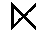Example: The relational algebra expression which I would here write as

PROJECTNamn ( SELECTMedlemsnummer < 3 ( Medlem ) )

should actually be written

## Cartesian product

The cartesian product of two tables combines each row in one table with each row in the other table.

Example: The table E (for EMPLOYEE)

enrenamedept
1BillA
2SarahC
3JohnA

Example: The table D (for DEPARTMENT)

dnrdname
AMarketing
BSales
CLegal

SQLResultRelational algebra
```select *
from E, D
```
enrenamedeptdnrdname
1BillAAMarketing
1BillABSales
1BillACLegal
2SarahCAMarketing
2SarahCBSales
2SarahCCLegal
3JohnAAMarketing
3JohnABSales
3JohnACLegal
E X D

• Seldom useful in practice.
• Usually an error.
• Can give a huge result.

## Join (sometimes called "inner join")

The cartesian product example above combined each employee with each department. If we only keep those lines where the dept attribute for the employee is equal to the dnr (the department number) of the department, we get a nice list of the employees, and the department that each employee works for:

SQLResultRelational algebra
```select *
from E, D
where dept = dnr
```
enrenamedeptdnrdname
1BillAAMarketing
2SarahCCLegal
3JohnAAMarketing
SELECTdept = dnr (E X D)

or, using the equivalent join operation

E JOINdept = dnr D

• A very common and useful operation.
• Equivalent to a cartesian product followed by a select.
• Inside a relational DBMS, it is usually much more efficient to calculate a join directly, instead of calculating a cartesian product and then throwing away most of the lines.
• Note that the same SQL query can be translated to several different relational algebra expressions, which all give the same result.
• If we assume that these relational algebra expressions are executed, inside a relational DBMS which uses relational algebra operations as its lower-level internal operations, different relational algebra expressions can take very different time (and memory) to execute.

## Natural join

A normal inner join, but using the join condition that columns with the same names should be equal. Duplicate columns are removed.

## Renaming tables and columns

Example: The table E (for EMPLOYEE)

nrnamedept
1BillA
2SarahC
3JohnA

Example: The table D (for DEPARTMENT)

nrname
AMarketing
BSales
CLegal

We want to join these tables, but:

• Several columns in the result will have the same name (nr and name).
• How do we express the join condition, when there are two columns called nr?

Solutions:

• Rename the attributes, using the rename operator.
• Keep the names, and prefix them with the table name, as is done in SQL. (This is somewhat unorthodox.)
SQLResultRelational algebra
```select *
from E as E(enr, ename, dept),
D as D(dnr, dname)
where dept = dnr
```
enrenamedeptdnrdname
1BillAAMarketing
2SarahCCLegal
3JohnAAMarketing
(RENAME(enr, ename, dept)(E)) JOINdept = dnr (RENAME(dnr, dname)(D))
```select *
from E, D
where dept = D.nr
```
nrnamedeptnrname
1BillAAMarketing
2SarahCCLegal
3JohnAAMarketing
E JOINdept = D.nr D

You can use another variant of the renaming operator to change the name of a table, for example to change the name of E to R. This is necessary when joining a table with itself (see below).

RENAMER(E)
A third variant lets you rename both the table and the columns:
RENAMER(enr, ename, dept)(E)

## Aggregate functions

Example: The table E (for EMPLOYEE)

nrnamesalarydept
1John100A
5Sarah300C
7Tom100A
12AnnenullC

SQLResultRelational algebra
```select sum(salary)
from E
```
sum
500
Fsum(salary)(E)

Note:

• Duplicates are not eliminated.
• Null values are ignored.
SQLResultRelational algebra
```select count(salary)
from E
```
Result:
count
3
Fcount(salary)(E)
```select count(distinct salary)
from E
```
Result:
count
2
Fcount(salary)(PROJECTsalary(E))

You can calculate aggregates "grouped by" something:

SQLResultRelational algebra
```select sum(salary)
from E
group by dept
```
deptsum
A200
C300
deptFsum(salary)(E)

Several aggregates simultaneously:

SQLResultRelational algebra
```select sum(salary), count(*)
from E
group by dept
```
deptsumcount
A2002
C3001
deptFsum(salary), count(*)(E)

Standard aggregate functions: sum, count, avg, min, max

## Hierarchies

Example: The table E (for EMPLOYEE)

nrnamemgr
1Gretchennull
2Bob1
5Anne2
6John2
3Hulda1
4Hjalmar1
7Usama4

Going up in the hierarchy one level: What's the name of John's boss?

SQLResultRelational algebra
```select b.name
from E p, E b
where p.mgr = b.nr
and p.name = "John"
```
name
Bob
PROJECTbname ([SELECTpname = "John"(RENAMEP(pnr, pname, pmgr)(E))] JOINpmgr = bnr [RENAMEB(bnr, bname, bmgr)(E)])

or, in a less wide-spread notation

PROJECTb.name ([SELECTname = "John"(RENAMEP(E))] JOINp.mgr = b.nr [RENAMEB(E)])

or, step by step

P <- RENAMEP(pnr, pname, pmgr)(E)
B <- RENAMEB(bnr, bname, bmgr)(E)
J <- SELECTname = "John"(P)
C <- J JOINpmgr = bnr B
R <- PROJECTbname(C)

• We are joining E with itself, both in the SQL query and in the relational algebra expression: it's like joining two tables with the same name and the same attribute names.
• Therefore, some renaming is required.
• RENAMEP(E) JOIN... RENAMEB(E) is a start, but then we still have the same attribute names.

Going up in the hierarchy two levels: What's the name of John's boss' boss?

SQLResultRelational algebra
```select ob.name
from E p, E b, E ob
where b.mgr = ob.nr
where p.mgr = b.nr
and p.name = "John"
```
name
Gretchen
PROJECTob.name (([SELECTname = "John"(RENAMEP(E))] JOINp.mgr = b.nr [RENAMEB(E)]) JOINb.mgr = ob.nr [RENAMEOB(E)])

or, step by step

P <- RENAMEP(pnr, pname, pmgr)(E)
B <- RENAMEB(bnr, bname, bmgr)(E)
OB <- RENAMEOB(obnr, obname, obmgr)(E)
J <- SELECTname = "John"(P)
C1 <- J JOINpmgr = bnr B
C2 <- C1 JOINbmgr = bbnr OB
R <- PROJECTobname(C2)

## Recursive closure

Both one and two levels up: What's the name of John's boss, and of John's boss' boss?

SQLResultRelational algebra
```(select b.name ...)
union
(select ob.name ...)
```
name
Bob
Gretchen
(...) UNION (...)

Recursively: What's the name of all John's bosses? (One, two, three, four or more levels.)

• Not possible in (conventional) relational algebra, but a special operation called transitive closure has been proposed.
• Not possible in (standard) SQL (SQL2), but in SQL3, and using SQL + a host language with loops or recursion.

## Outer join

Example: The table E (for EMPLOYEE)

enrenamedept
1BillA
2SarahC
3JohnA

Example: The table D (for DEPARTMENT)

dnrdname
AMarketing
BSales
CLegal

List each employee together with the department he or she works at:

SQLResultRelational algebra
```select *
from E, D
where edept = dnr
```

or, using an explicit join
```select *
from (E join D on edept = dnr)
```
enrenamedeptdnrdname
1BillAAMarketing
2SarahCCLegal
3JohnAAMarketing
E JOINedept = dnr D

No employee works at department B, Sales, so it is not present in the result. This is probably not a problem in this case. But what if we want to know the number of employees at each department?

SQLResultRelational algebra
```select dnr, dname, count(*)
from E, D
where edept = dnr
group by dnr, dname
```

or, using an explicit join
```select dnr, dname, count(*)
from (E join D on edept = dnr)
group by dnr, dname
```
dnrdnamecount
AMarketing2
CLegal1
dnr, dnameFcount(*)(E JOINedept = dnr D)

No employee works at department B, Sales, so it is not present in the result. It disappeared already in the join, so the aggregate function never sees it. But what if we want it in the result, with the right number of employees (zero)?

Use a right outer join, which keeps all the rows from the right table. If a row can't be connected to any of the rows from the left table according to the join condition, null values are used:

SQLResultRelational algebra
```select *
from (E right outer join D on edept = dnr)
```
enrenamedeptdnrdname
1BillAAMarketing
2SarahCCLegal
3JohnAAMarketing
nullnullnullBSales
E RIGHT OUTER JOINedept = dnr D
```select dnr, dname, count(*)
from (E right outer join D on edept = dnr)
group by dnr, dname
```
dnrdnamecount
AMarketing2
BSales1
CLegal1
dnr, dnameFcount(*)(E RIGHT OUTER JOINedept = dnr D)
```select dnr, dname, count(enr)
from (E right outer join D on edept = dnr)
group by dnr, dname
```
dnrdnamecount
AMarketing2
BSales0
CLegal1
dnr, dnameFcount(enr)(E RIGHT OUTER JOINedept = dnr D)

Join types:

• JOIN = "normal" join = inner join
• LEFT OUTER JOIN = left outer join
• RIGHT OUTER JOIN = right outer join
• FULL OUTER JOIN = full outer join

## Outer union

Outer union can be used to calculate the union of two relations that are partially union compatible. Not very common.

Example: The table R

AB
12
34

Example: The table S

BC
45
67

The result of an outer union between R and S:

ABC
12null
345
null67

## Division

Who works on (at least) all the projects that Bob works on?

## Semijoin

A join where the result only contains the columns from one of the joined tables. Useful in distributed databases, so we don't have to send as much data over the network.

## Update

To update a named relation, just give the variable a new value. To add all the rows in relation N to the relation R:

R <- R UNION N